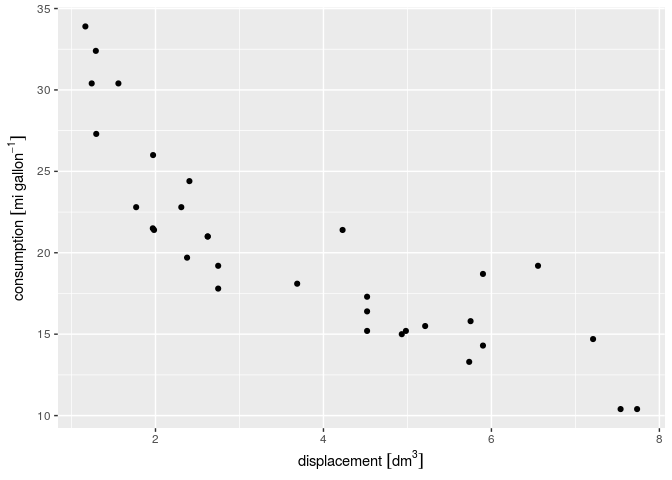This blog post concerns the development version of units, installed by

``````devtools::install_github("edzer/units")
``````

Have you ever tried to properly add measurement units to R plots? It might go like this:

``````xlab = parse(text = "temperature ~~ group('[', degree * C, ']')")
ylab = parse(text = "speed ~~ group('[', m * ~~ s^-1, ']')")
par(mar = par("mar") + c(0, .3, 0, 0)) # avoids cutting of superscript
plot(3 + 1:10 + 2 * rnorm(10), xlab = xlab, ylab = ylab)
``````The main observation is, of course that it can be done. However,

• it looks geeky, and not quite intuitive
• you would typically postpone this work to just before submitting the paper, or during review
• you need this so infrequently that you tend to forget how it works.

Although well-written help is found in `?plotmath`, all three observations cause frustration.

The original paper desribing `plotmath` is by Paul Murrell and Ross Ihaka. R core member Paul Murrell also wrote package `grid`, part of base R. Few people use it directly, but without it ggplot2 or lattice could not exist.

## Automatic unit handling

The new units CRAN package now makes working with units

• easier
• automatic, and
• less error-prone

Here is an example using `mtcars`. First, we specify the imperial units to those known in the udunits2 database:

``````library(units)
gallon = make_unit("gallon")
consumption = mtcars\$mpg * with(ud_units, mi/gallon)
displacement = mtcars\$disp * ud_units[["in"]]^3
``````

For `displacement`, we cannot use the normal lookup in the database

``````displacement = mtcars\$disp * with(ud_units, in)
``````

because `in` (inch) is also a reserved word in R.

We convert these values to SI units by

``````units(displacement) = with(ud_units, cm^3)
units(consumption) = with(ud_units, km/l)
consumption[1:5]

## Units: km/l
##  8.928017 8.928017 9.693276 9.098075 7.950187
``````

## Automatic measurement units in axis labels

We can plot these numeric variabes of type `units` by

``````par(mar = par("mar") + c(0, .1, 0, 0)) # avoids cutting of brackets at lhs
plot(displacement, consumption)
``````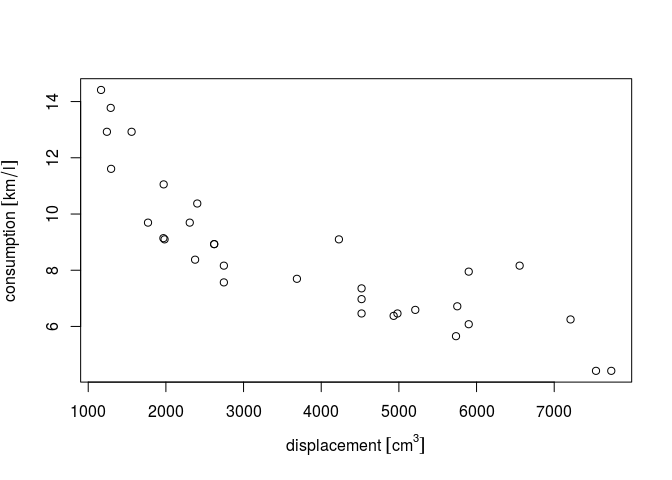The units automatically appear in axis labels! If we want to have negative power instead of division bars, we can set a global option

``````units_options(negative_power = TRUE) # division becomes ^-1
``````

Expressions such as

``````1/displacement [1:10]

## Units: cm^-3
##   0.0003813984 0.0003813984 0.0005650347 0.0002365261 0.0001695104
##   0.0002712166 0.0001695104 0.0004159764 0.0004334073 0.0003641035
``````

automatically convert units, which also happens in plots (note the converted units symbols):

``````par(mar = par("mar") + c(0, .3, 0, 0))
plot(1/displacement, 1/consumption)
``````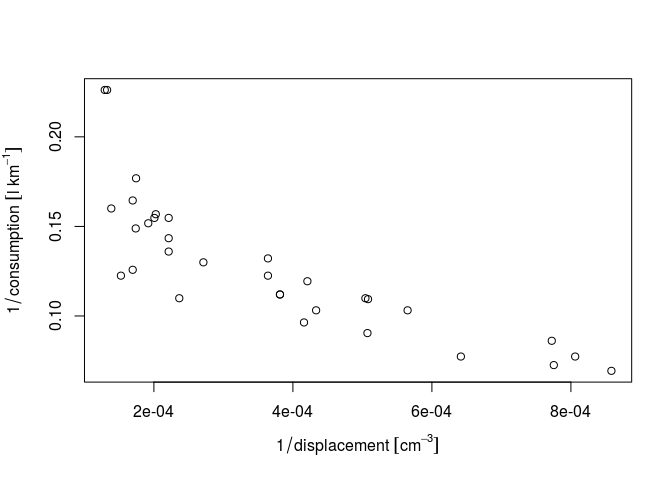## How to do this with ggplot?

We can of course plot these data by dropping units:

``````library(ggplot2)
ggplot() + geom_point(aes(x = as.numeric(displacement),
y = as.numeric(consumption)))
``````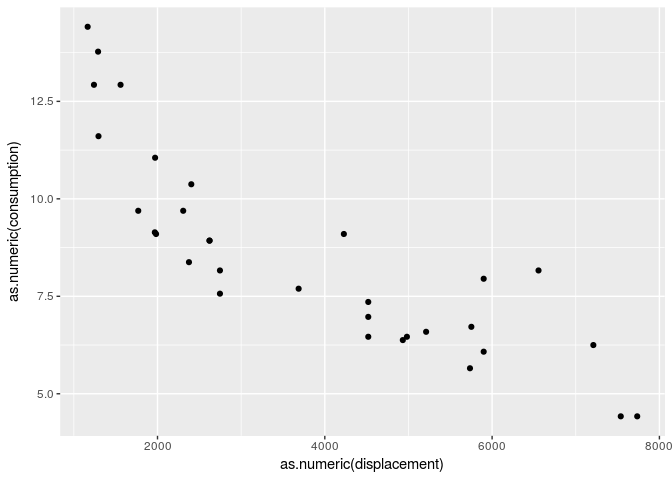but that doesn’t show us units. Giving the units as variables gives an error:

``````ggplot() + geom_point(aes(x = displacement, y = consumption))

## Don't know how to automatically pick scale for object of type units. Defaulting to continuous.
## Don't know how to automatically pick scale for object of type units. Defaulting to continuous.

## Error in Ops.units(x, range): both operands of the expression should be "units" objects
``````(I could make that error go away by letting `units` drop the requirement that in a comparison both sides should have compatible units, which of course would be wrong.)

We can then go all the way with

``````ggplot() + geom_point(aes(x = as.numeric(displacement),
y = as.numeric(consumption))) +
xlab(make_unit_label("displacement", displacement)) +
ylab(make_unit_label("consumption", consumption))
``````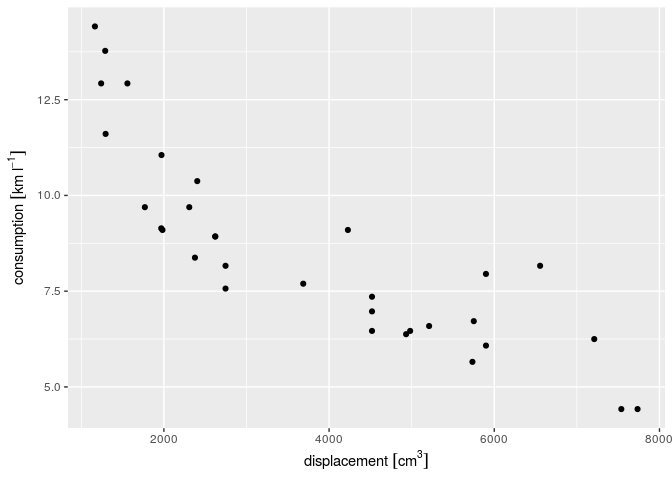which at least doesn’t cut off the left label, but feels too convoluted and error-prone.

Oh ggplot gurus, who can help us out, here? How can we obtain that last plot by

``````ggplot() + geom_point(aes(x = displacement, y = consumption))
``````

?

## Update of Dec 2, 2016

Thanks to ggguru Thomas Lin Pedersen, automatic units in axis labels of ggplots are now provided by CRAN package ggforce:

``````library(ggforce)
ggplot() + geom_point(aes(x = displacement, y = consumption))
``````and see this vignette for more examples. In addition to printing units in default axes labels, it allows for on-the-fly unit conversion in ggplot expressions:

``````dm = with(ud_units, dm)
gallon = with(ud_units, gallon)
mi = with(ud_units, mi)
ggplot() + geom_point(aes(x = displacement, y = consumption)) +
scale_x_unit(unit = dm^3) + scale_y_unit(unit = mi/gallon)
``````